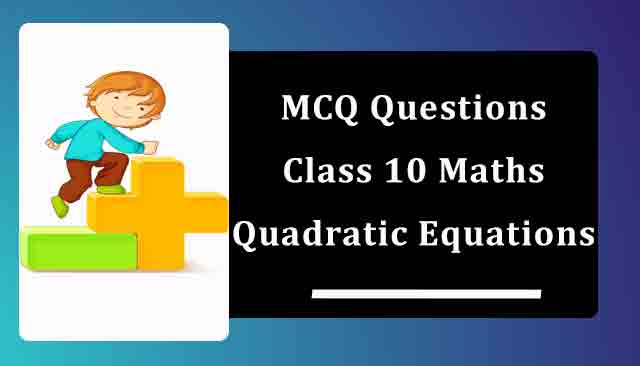# MCQ Questions for Class 10 Maths Chapter 4 Quadratic Equations with Answers

Below you will find NCERT MCQ Questions for Class 10 Maths Chapter 4 Quadratic Equations with Answers which is very helpful during the preparation of examinations. These MCQ Online Test are one marks questions which will give you overview of the chapter in no time. One should try to understand Class 10 MCQ Questions as it is based on latest exam pattern released by CBSE.

Also, students can check NCERT Solutions for Class 10 Maths Chapter 4 for improving their marks and have good understanding of the chapter.## Chapter 4 Quadratic Equations MCQ Questions for Class 10 Maths with Answers

1. Which of the following are the roots of the quadratic equation, x2 - 9x + 20 = 0 by factorisation?
(a) 3,4
(b) 4, 5
(c) 5, 6
(d) 6, 7
Solution (b) 4, 5

2. Which of the following equations has 2 as a root?
(a) x2 – 4x + 5 = 0
(b) x2 + 3x – 12 = 0
(c) 2x2 – 7x + 6 = 0
(d) 3x2 – 6x – 2 = 0
Solution (c) 2x2 – 7x + 6 = 0

3. Values of k for which the quadratic equation  2x2 – kx + k = 0 has equal roots is:
(a) 0 only
(b) 4
(c) 8 only
(d) 0, 8
Solution (d) 0, 8

3. The sum of areas of two squares is 468m2. If the difference of their perimeters is 24m, then the sides of the two squares are:​
(a) 12m and 18m
(b) 24m and 28
(c) 6m and 12m
(d) 18m and 24m
Solution (a) 12m and 18m

4. If ‘a’ and ‘b’ are the roots of the equation x2+ax+b = 0, then a+b =
(a) – 2
(b) a
(c) – a
(d) 2
Solution (c) – a

5. The rooks of the equation x2+ 7x + 10 = 0 are:
(a) – 5, – 2
(b) 5, 2
(c) 5, – 2
(d) – 5, 2
Solution (a) – 5, – 2

6. The equation x2 – px + q = 0 p, q e R has no real roots if :
(a) p2 > 4q
(b) p2 < 4q
(c) p2 = 4q
(d) None of these
Solution (b) p2 < 4q

7. If the roots of px2 + qx + 2 = 0 are reciprocal of each other, then
(a) P = 0
(b) p = -2
(c) p = ±2
(d) p = 2
Solution (d) p = 2

8. Root of the equation x2 - 0.09 = 0 is
(a) 0.3
(b) 0.03
(c) no root
(d) none of these
Solution (a) 0.3

9. Which of the following equations has - 1 as a root?
(a) x2 + 3x - 10 = 0
(b) x2 - x - 12 = 0
(c) 3x2 - 2x - 5 = 0
(d) 9x2 + 24x + 16 = 0
Solution (c) 3x2 - 2x - 5 = 0

10. The common root of 2x2+x−6 = 0 and x2−3x−10 = 0 is
(a) – 2
(b) 5
(c) 3/2
(d) 2
Solution (a) – 2

11. The product of two successive integral multiples of 5 is 1050. Then the numbers are
(a) 30 and 35
(b) 25 and 30
(c) 35 and 40
(d) 25 and 42
Solution (a) 30 and 35

12. (x2+1)2 − x2 = 0 has
(a) no real roots
(b) 1 real roots
(c) 2 real roots
(d) 3 real roots
Solution (a) no real roots

13. What are the two consecutive even integers whose squares have sum 340?​
(a) 12 and 10
(b) –12 and –14
(c) 12 and 14
(d) Both (b) and (c)
Solution (d) Both (b) and (c)

14. If the equation x2 - kx +9 = 0 does not possess real roots, then
(a) -6 < k < 6
(b) k > 6
(c) k < -6
(d) k = ±6
Solution (a) -6 < k < 6

15. The equation 4x2 = 4x has following solution/solutions
(a) 1
(b) -1, 0
(c) 1, -1
(d) 0, 1
Solution (d) 0, 1

16. Divide 16 into two parts such that twice the square of the larger part exceeds the square of the smaller part by 164.​
(a) 14 and 2
(b) 11 and 5
(c) 12 and 4
(d) 10 and 6
Solution (d) 10 and 6

17. 5x2+8x+4 = 2x2+4x+6 is a
(a) linear equation
(b) constant
(c) cubic equation

18. Find the two consecutive odd positive integers, sum of whose square is 290.
(a) 15, 17
(b) 9, 11
(c) 13, 15
(d) 11, 13
Solution (d) 11, 13

19. (x2 + 1)2 - x2 = 0 has
(a) Four real roots
(b) Two real roots
(c) No real roots
(d) One real root
Solution (c) No real roots

20. Which of the following equations has 2 as a root?
(a) x2 - 4x + 5 = 0
(b) x2 + 3x - 12 = 0
(c) 2x2 - 7x + 6 = 0
(d) 3x2 - 6x - 2 = 0
Solution (c) 2x2 - 7x + 6 = 0

21. If α, β are roots of the equation x2 + 5x + 5 = 0, then equation whose roots are α + 1 and β + 1 is
(a) x2 + 5x - 5 = 0
(b) x2 + 3x + 5 = 0
(c) x2 + 3x + 1 = 0
(d) none of these
Solution (c) x2 + 3x + 1 = 0

22. If x = 2 is a root of the quadratic equation 3x2 – px – 2 = 0, then the value of ‘p’ is
(a) 1
(b) 5
(c) 3
(d) 0
Solution (b) 5